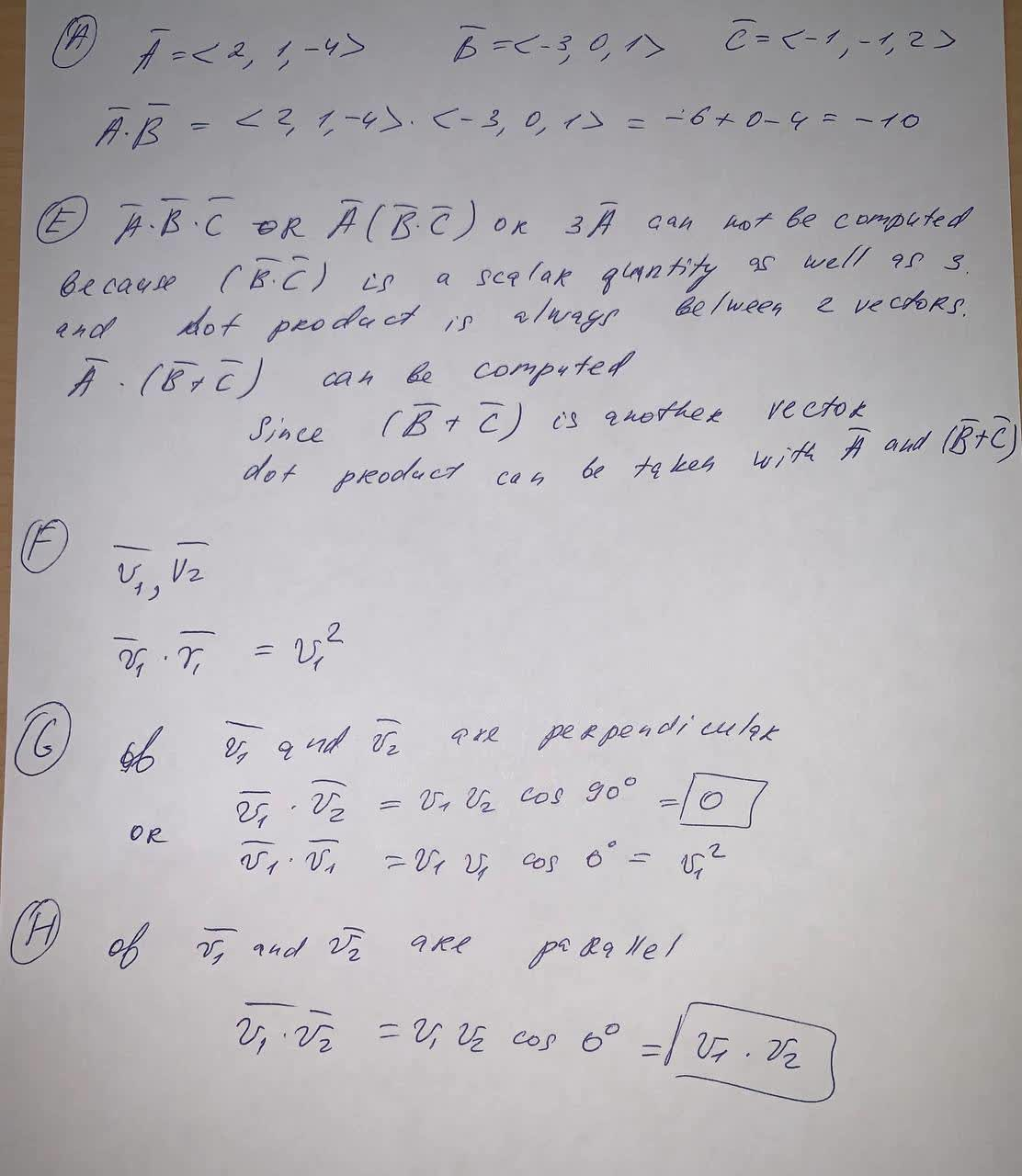# Let vectors \bar{A} =(2,1,-4), \bar{B} =(-3,0,1), \text{ and } \bar{C} =(-1,-1,2) Calculate the following: A)\bar{A} \cdot \bar{B}? E)Which of the folAmari Flowers 2021-06-03 Answered
Let vectors
Calculate the following:
A)$\overline{A}\cdot \overline{B}$?
E)Which of the following can be computed?
$\overline{A}\cdot \overline{B}\cdot \overline{C}$
$\overline{A}\left(\overline{B}\cdot \overline{C}\right)$
$\overline{A}\left(\overline{B}+\overline{C}\right)$
$3\overline{A}$
F)Express your answer in terms of ${v}_{1}$
G) If are perpendicular?
H) If are parallel?
You can still ask an expert for help

• Questions are typically answered in as fast as 30 minutes

Solve your problem for the price of one coffee

• Math expert for every subject
• Pay only if we can solve itunett###### Not exactly what you’re looking for?Jeffrey Jordon

A)

$\stackrel{―}{A}\cdot \stackrel{―}{B}=<2,1,-4>\cdot <-3,0,1>=-6+0-4=-10$

E) $\stackrel{―}{A}\cdot \stackrel{―}{B}\cdot \stackrel{―}{C}$ or $\stackrel{―}{A}\left(\stackrel{―}{B}\cdot \stackrel{―}{C}\right)$ or $3\stackrel{―}{A}$ can not be computed because $\left(\stackrel{―}{B}\cdot \stackrel{―}{C}\right)$ is a scalar quatitty as well as 3 and dot product is always belween 2 vectors.

$\stackrel{―}{A}\cdot \left(\stackrel{―}{B}+\stackrel{―}{C}\right)$ can be computed

Since $\left(\stackrel{―}{B}+\stackrel{―}{C}\right)$ is another vector dot product can be taken with $\stackrel{―}{A}$ and $\left(\stackrel{―}{B}+\stackrel{―}{C}\right)$

F) $\stackrel{―}{{v}_{1}},\stackrel{―}{{v}_{2}}$

$\stackrel{―}{{v}_{1}}\cdot \stackrel{―}{{v}_{1}}={v}_{1}^{2}$

G) $\stackrel{―}{{v}_{1}}$ and $\stackrel{―}{{v}_{2}}$ are perpendicular

$\stackrel{―}{{v}_{1}}\cdot \stackrel{―}{{v}_{2}}={v}_{1}{v}_{2}\mathrm{cos}{90}^{\circ }=0$

$\stackrel{―}{{v}_{1}}\cdot \stackrel{―}{{v}_{1}}={v}_{1}{v}_{1}\mathrm{cos}{0}^{\circ }={v}_{1}^{2}$

of $\stackrel{―}{{v}_{1}}$ and $\stackrel{―}{{v}_{2}}$ are parallel

$\stackrel{―}{{v}_{1}}\cdot \stackrel{―}{{v}_{2}}={v}_{1}{v}_{2}\mathrm{cos}{0}^{\circ }={v}_{1}\cdot {v}_{2}$